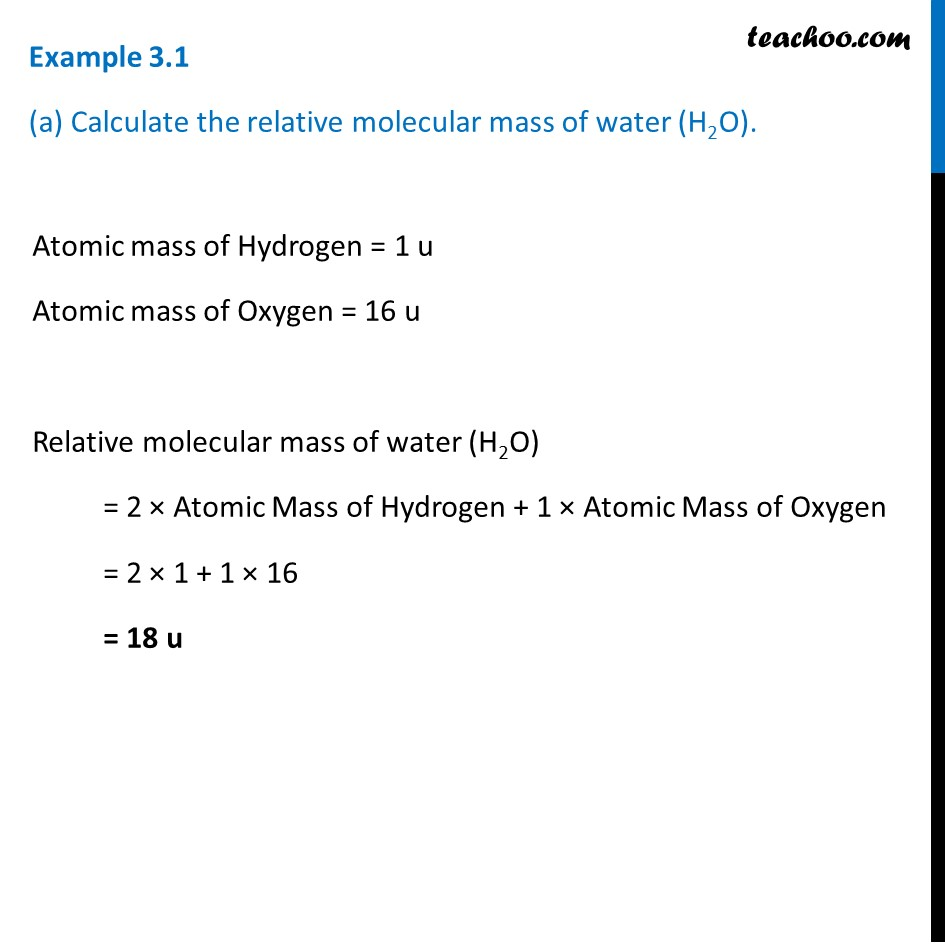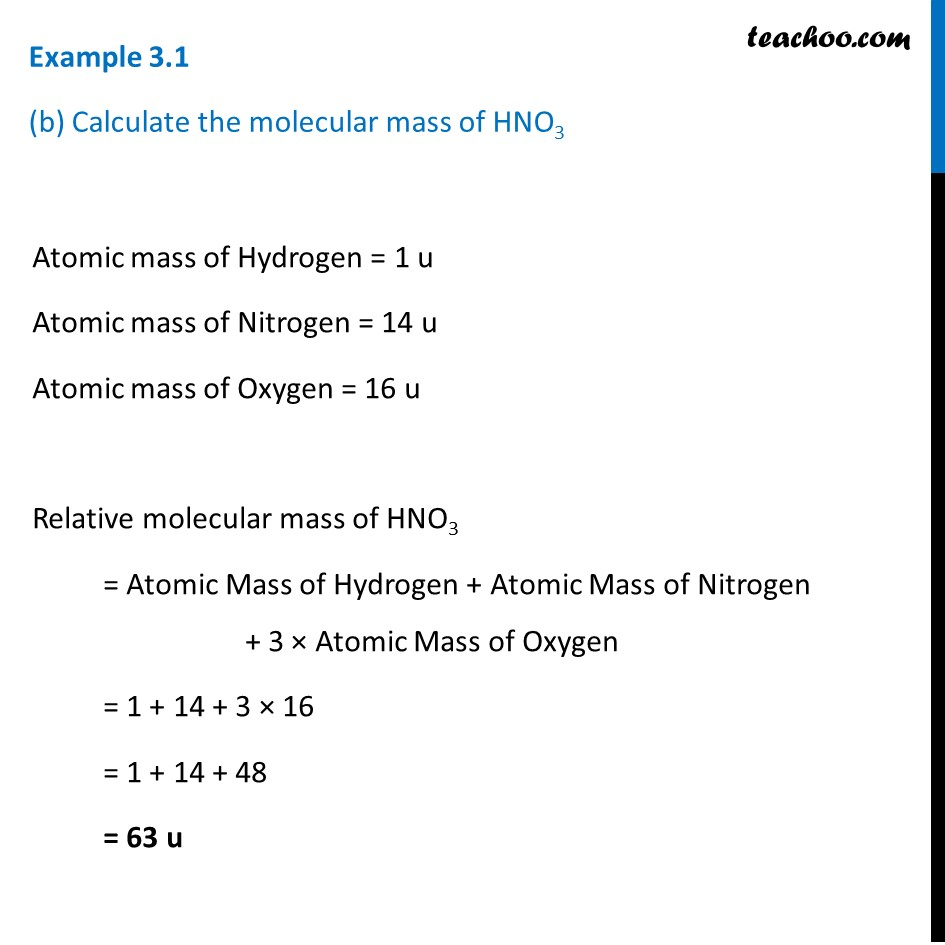Examples from NCERT Book

Class 9
Chapter 3 Class 9 - Atoms And MoleculesLearn in your speed, with individual attention - Teachoo Maths 1-on-1 Class

### Transcript

Example 3.1 (a) Calculate the relative molecular mass of water (H2O). Atomic mass of Hydrogen = 1 u Atomic mass of Oxygen = 16 u Relative molecular mass of water (H2O) = 2 × Atomic Mass of Hydrogen + 1 × Atomic Mass of Oxygen = 2 × 1 + 1 × 16 = 18 u Example 3.1 (b) Calculate the molecular mass of HNO3 Atomic mass of Hydrogen = 1 u Atomic mass of Nitrogen = 14 u Atomic mass of Oxygen = 16 u Relative molecular mass of HNO3 = Atomic Mass of Hydrogen + Atomic Mass of Nitrogen + 3 × Atomic Mass of Oxygen = 1 + 14 + 3 × 16 = 1 + 14 + 48 = 63 u